tags:机器学习

《Machine Learning in Action》—— 浅谈线性回归的那些事

• 线性回归，我们来谈谈小姐姐如何相亲
• 揭开梯度下降算法的神秘面纱
• 基于梯度下降算法实现线性回归拟合

一、线性回归，我们来谈谈小姐姐如何相亲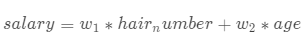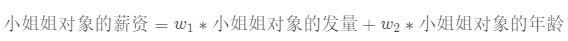二、揭开梯度下降算法的神秘面纱• 单变量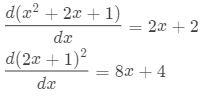• 多变量（以三个变量为例）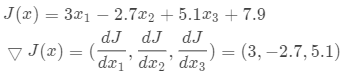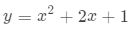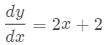①：x_new = x_old - learning_rate * gradient(x_old)

②：learning_rate

learning_rate在梯度下降算法中被称作为学习率或者说是步长，意味着我们可以通过learning_rate来控制每一步走的距离，其实就是不要走太快，从而错过了最低点。同时也要保证不要走的太慢，导致我们打到最低点需要花费大量的时间，也就是效率太低了，需要迭代很多次才能满足我们的需求。所以learning_rate的选择在梯度下降法中往往是很重要的！

1. 随机梯度下降算法（SGD，根据时间复杂度）
2. 批量梯度下降算法（BGD，根据数据量的大小）
3. 小批量梯度下降算法（MBGD，算法准确性）

三、基于梯度下降算法实现线性回归拟合

1943年，心理学家沃伦·麦卡洛克(Warren McCulloch)和数理逻辑学家沃尔特·皮茨(Walter Pitts)通过对生物神经元的研究，提出了模拟生物神经元机制的人工神经网络的数学模型，这一成果被美国神经学家弗兰克·罗森布拉特(Frank Rosenblatt)进一步发展成感知机(Perceptron)模型，这也是现代深度学习的基石。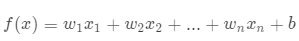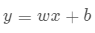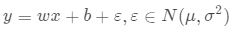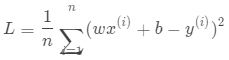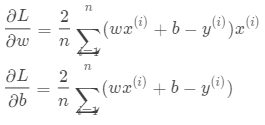• NumPy随机生成数据集，并通过Matplotlib初步观察数据的分布
"""
Author: Taoye
微信公众号: 玩世不恭的Coder
Explain: 用于生成样本数据
Return:
x_data: 数据样本的一个属性
y_data: 数据样本的另一个属性
"""

def establish_data(data_number):
x_data = np.random.uniform(-1010, data_number)
eps = np.random.normal(01, data_number)
y_data = x_data * 1.474 + 0.86 + eps
return x_data, y_data

if __name__ == "__main__":
x_data, y_data = establish_data(100)
from matplotlib import pyplot as plt
plt.scatter(x_data, y_data)
plt.show()

• 根据数据和当前的w、b值计算均方误差
"""
Author: Taoye
微信公众号: 玩世不恭的Coder
Explain: 计算均方误差
Parameters:
x_data: 数据样本的一个属性
y_data: 数据样本的另一个属性
w_now: 当前的w参数
b_now: 当前的b参数
Return:
mse_value: 均方误差值
"""

def calc_mse(x_data, y_data, w_now, b_now):
x_data, y_data = np.mat(x_data), np.mat(y_data)
_, data_number = x_data.shape
return np.power(w_now * x_data + b_now - y_data, 2).sum() / float(data_number)
• 单次对w、b参数进行更新迭代
"""
Author: Taoye
微信公众号: 玩世不恭的Coder
Explain: 更新迭代一次w、b
Parameters:
x_data: 数据样本的一个属性
y_data: 数据样本的另一个属性
w_now: 当前的w参数
b_now: 当前的b参数
learning_rate: 学习率
Return：
w_new: 更新迭代之后的w
b_new: 更新迭代之后的b
"""

def step_gradient(x_data, y_data, w_now, b_now, learning_rate):
x_data, y_data = np.mat(x_data), np.mat(y_data)
w = (w_now * x_data + b_now - y_data) * x_data.T * 2 / x_data.shape
b = (w_now * x_data + b_now - y_data).sum() * 2 / x_data.shape
return w_now - w * learning_rate, b_now - b * learning_rate
• 多次迭代更新w、b（外循环）
"""
Author: Taoye
微信公众号: 玩世不恭的Coder
Explain: 多次迭代更新w、b（外循环）
Parameters:
x_data: 数据样本的一个属性
y_data: 数据样本的另一个属性
starting_w: 初始的w参数
starting_b: 初试的b参数
learning_rate: 学习率
max_iter：最大迭代次数
Return：
w：得到的最终w
b: 得到的最终b
loss_list： 每次迭代计算的损失值
"""

def gradient_descent(x_data, y_data, starting_b, starting_w, learning_rate, max_iter):
b, w = starting_b, starting_w
loss_list = list()
for step in range(max_iter):
w, b = step_gradient(x_data, y_data, w, b, learning_rate)
loss = calc_mse(x_data, y_data, w, b)
loss_list.append(loss)
return w, b, np.array(loss_list)
• 拟合结果的可视化
"""
Author: Taoye
微信公众号: 玩世不恭的Coder
Explain: 拟合结果的可视化
Parameters:
x_data: 数据样本的一个属性
y_data: 数据样本的另一个属性
w: 拟合得到的模型w参数
b: 拟合得到的模型b参数
loss_list: 每次更新迭代得到的损失函数的值
"""

def plot_result(x_data, y_data, w, b, loss_list):
from matplotlib import pyplot as plt
%matplotlib inline

plt.subplot(211)
plt.scatter(x_data, y_data)
x_line_data = np.linspace(-10101000)
y_line_data = x_line_data * w + b
plt.plot(x_line_data, y_line_data, "--", color = "red")

plt.subplot(212)
plt.plot(np.arange(loss_list.shape), loss_list)
plt.show()

import numpy as np

"""
Author: Taoye
微信公众号: 玩世不恭的Coder
Explain: 用于生成样本数据
Return:
x_data: 数据样本的一个属性
y_data: 数据样本的另一个属性
"""

def establish_data(data_number):
x_data = np.random.uniform(-1010, data_number)
eps = np.random.normal(01, data_number)
y_data = x_data * 1.474 + 0.86 + eps
return x_data, y_data

"""
Author: Taoye
微信公众号: 玩世不恭的Coder
Explain: 计算均方误差
Parameters:
x_data: 数据样本的一个属性
y_data: 数据样本的另一个属性
w_now: 当前的w参数
b_now: 当前的b参数
Return:
mse_value: 均方误差值
"""

def calc_mse(x_data, y_data, w_now, b_now):
x_data, y_data = np.mat(x_data), np.mat(y_data)
_, data_number = x_data.shape
return np.power(w_now * x_data + b_now - y_data, 2).sum() / float(data_number)

"""
Author: Taoye
微信公众号: 玩世不恭的Coder
Explain: 更新迭代一次w、b
Parameters:
x_data: 数据样本的一个属性
y_data: 数据样本的另一个属性
w_now: 当前的w参数
b_now: 当前的b参数
learning_rate: 学习率
Return：
w_new: 更新迭代之后的w
b_new: 更新迭代之后的b
"""

def step_gradient(x_data, y_data, w_now, b_now, learning_rate):
x_data, y_data = np.mat(x_data), np.mat(y_data)
w = (w_now * x_data + b_now - y_data) * x_data.T * 2 / x_data.shape
b = (w_now * x_data + b_now - y_data).sum() * 2 / x_data.shape
return w_now - w * learning_rate, b_now - b * learning_rate

"""
Author: Taoye
微信公众号: 玩世不恭的Coder
Explain: 多次迭代更新w、b（外循环）
Parameters:
x_data: 数据样本的一个属性
y_data: 数据样本的另一个属性
starting_w: 初始的w参数
starting_b: 初试的b参数
learning_rate: 学习率
max_iter：最大迭代次数
Return：
w：得到的最终w
b: 得到的最终b
loss_list： 每次迭代计算的损失值
"""

def gradient_descent(x_data, y_data, starting_b, starting_w, learning_rate, max_iter):
b, w = starting_b, starting_w
loss_list = list()
for step in range(max_iter):
w, b = step_gradient(x_data, y_data, w, b, learning_rate)
loss = calc_mse(x_data, y_data, w, b)
loss_list.append(loss)
return w, b, np.array(loss_list)

"""
Author: Taoye
微信公众号: 玩世不恭的Coder
Explain: 拟合结果的可视化
Parameters:
x_data: 数据样本的一个属性
y_data: 数据样本的另一个属性
w: 拟合得到的模型w参数
b: 拟合得到的模型b参数
loss_list: 每次更新迭代得到的损失函数的值
"""

def plot_result(x_data, y_data, w, b, loss_list):
from matplotlib import pyplot as plt
%matplotlib inline

plt.subplot(211)
plt.scatter(x_data, y_data)
x_line_data = np.linspace(-10101000)
y_line_data = x_line_data * w + b
plt.plot(x_line_data, y_line_data, "--", color = "red")

plt.subplot(212)
plt.plot(np.arange(loss_list.shape), loss_list)
plt.show()

if __name__ == "__main__":
x_data, y_data = establish_data(100)
w, b, loss_list = gradient_descent(x_data, y_data, 000.011000)
plot_result(x_data, y_data, w, b, loss_list)

 《机器学习实战》：Peter Harrington 人民邮电出版社
 《TensorFlow深度学习》：龙龙老师
梯度下降算法原理讲解：https://blog.csdn.net/qq_41800366/article/details/86583789

posted @ 2020-12-05 14:48  玩世不恭的Coder  阅读(6320)  评论(12编辑  收藏  举报